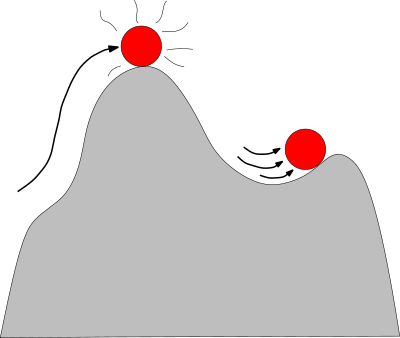Structural Bioinformatics Library Template C++ / Python API for developping structural bioinformatics applications.
Molecular_potential_energy

Computation of the potential energy of a molecule Reference ManualUser Manual . Authors: F. Cazals and A. Chevallier and T. DreyfusMain classes:

Defines iterator over the bonds of a covalent structure.

Defines iterator over the bond angles of a covalent structure.

Defines iterator over the dihedral angles of a covalent structure.

Defines iterator over the improper angles of a covalent structure.

Defines the standard term associated to the bond length contribution.

Defines the standard term associated to the bond angle contribution.

Defines the Urey-Bradley term associated to a bond angle.

Defines the harmonic term associated to the proper dihedral angle contribution.

Defines the periodic term associated to the proper dihedral angle contribution.

Defines the Fourier term associated to the proper dihedral angle contribution.

Defines the Ryckaert_Bellemans term associated to the proper dihedral angle contribution.

Defines the CMap term associated to a pair of proper dihedral angles.

Defines the standard term associated to the Lennard Jones contribution.

Defines a standard term associated to the electrostatic contribution.

Contribution of an energy term to the potential energy function.

Contribution of all bonded energy terms to the potential energy function.

Potential energy function adding up all the input contributions (C++11 only).

Defines the gradient associated to the bond angle.

Defines the gradient associated to the torsion angle.

Defines the standard gradient associated to the bond length contribution.

Defines the standard gradient associated to the bond angle contribution.

Defines the harmonic gradient associated to the proper dihedral angle contribution.

Defines the periodic gradient associated to the proper dihedral angle contribution.

Defines the Fourier gradient associated to the proper dihedral angle contribution.

Defines the Ryckaert_Bellemans gradient associated to the proper dihedral angle contribution.

Defines the gradient associated to the torsion angle contribution in a linear structure.

Defines the standard gradient associated to the Lennard Jones contribution.

Defines a standard gradient associated to the electrostatic contribution.

Contribution of an energy gradient term to the potential energy gradient function.

Sum of the contributions of an energy gradient term to the potential energy gradient function.

Computes numerically the gradient of a potential energy function.

Defines the term associated to the torsion angle contribution in a linear structure.